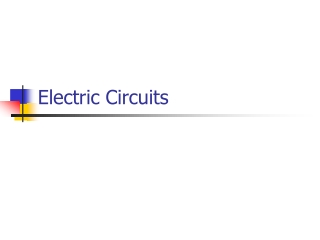DownloadDownload PresentationElectric Circuits

Electric Circuits

Télécharger la présentationElectric Circuits

- - - - - - - - - - - - - - - - - - - - - - - - - - - E N D - - - - - - - - - - - - - - - - - - - - - - - - - - -
Presentation Transcript

1. Electric Circuits

2. Electric Circuits • Provide one or more complete paths for current to follow. All circuits have: • A source of potential difference • A load that uses electrical energy • A conducting path connecting them (wires) • A switch can be used to break or complete the path (on/off)

3. Electric Circuits • Closed Circuit: A complete conducting path. Current flows • Open Circuit: The path is broken. Current stops. • Short Circuit: Circuit with no load. Current is very high, can cause overheating of wires and is very dangerous.

4. Series Circuit • Provides only one path for current. • All devices carry the same current. • Voltage drop across each device adds to give the total voltage for the circuit. • Total resistance is the sum of the individual resistors (Req = R1 + R2)

5. Parallel Circuit • Provides two or more paths (branches) for current to follow. • Sum of currents in each branch equals the total current in the circuit. • Voltage drop across all devices is the same. • 1/Req = 1/R1 + 1/R2